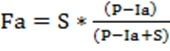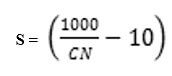# How can estimate the rainfall excess from the design storm

The approach is SCS CN Method. In this method, According to the SCS method, the total rainfall (P) is divided into three parts: initial abstraction (Ia), continuous abstraction (Fa) and excess rainfall (Pe). Initial abstraction is the amount of rainfall that is used for satisfying surface depressions, and never leaves the watershed. It is calculated as 20% of the total maximum storage or retention (S) of the watershed. Therefore, Ia = 0.2S. After this initial abstraction is satisfied, the remaining amount of rainfall is used to satisfy remaining soil storage as continuous abstraction (Fa). The expression to compute Fa by using the total amount of rainfall (P) and the maximum retention (S) is given by the following equation:where, P, Fa, S and Ia have the same unit of length [L]. Usually total rainfall (P) is known, and S is computed by using the CN (assumed to be given for this exercise) as shown in the equation below:Where S is in inches. If the rainfall data is given in some other units, S needs to be converted to those units before using in the SCS equation. Essentially, the continuous abstraction term Fa gives the amount of rainfall that is lost as infiltration from the total rainfall. Where S is in inches. If the rainfall data is given in some other units, S needs to be converted to those units before using in the SCS equation.

Name: Hidden